• Nota de Estudos
• Rever Tópicos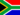No M. 0 0 The higher odds correspond to higher probability and low odds low probability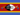Samkeliso R S. 0 0 odds express probability in ways that makes payouts easy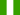Juliet N. 0 0 odds of lossing and probability of wining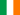Rachel N. 0 0 gambling uses odds to calculate probabilityCorey N. 0 0 Completely unnecessary and childish.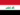Olga K. 0 0 Can somebody help me with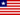Zayzay K. 0 0 odds of losing and probability of wining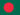Jahidul I. 0 0 Odds and Probabilities Odds and probabilities If there is a $p$ probability of something happening, then the odds can be considered the number of successes you expect to get for every failure on average. High odds correspond to high probabilities, low odds to low probabilities. To calculate the odds: $\displaystyle O=\frac{\text{proportion of successes}}{\text{proportion of failures}}=\frac{p}{1-p}$ To go from odds back to probabilities: $\displaystyle p=\frac{O}{1+O}$ While probabilities are bound to [0,1], odds are bound to [0,$\infty$) Let's take the titanic example. What was the overall odds of survival on the Titanic. 38% of passengers survived the Titanic. Therefore: Odds of survival$\displaystyle =\frac{0.38}{1-.38}=\frac{0.38}{0.62}=0.61$Jahidul I. 0 0 what is Basic Probability Formulas?
• Text Version### Você recebeu uma nova notificação

Clique aqui para visualizar todos eles